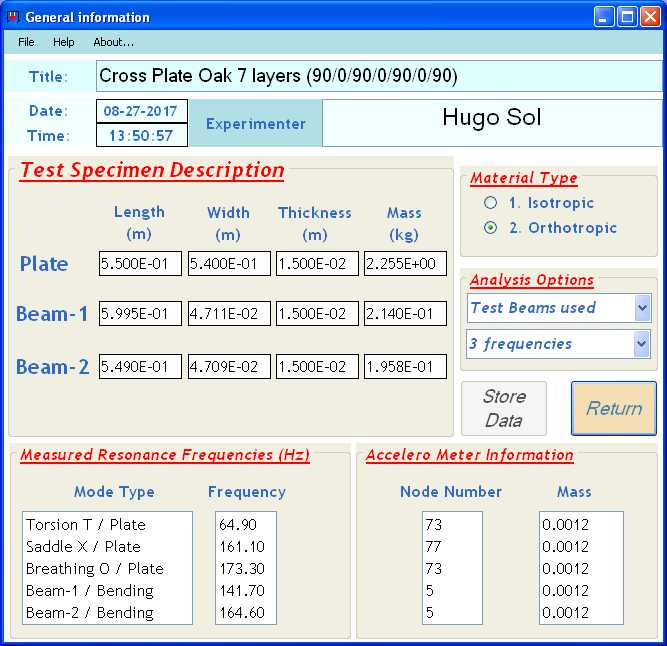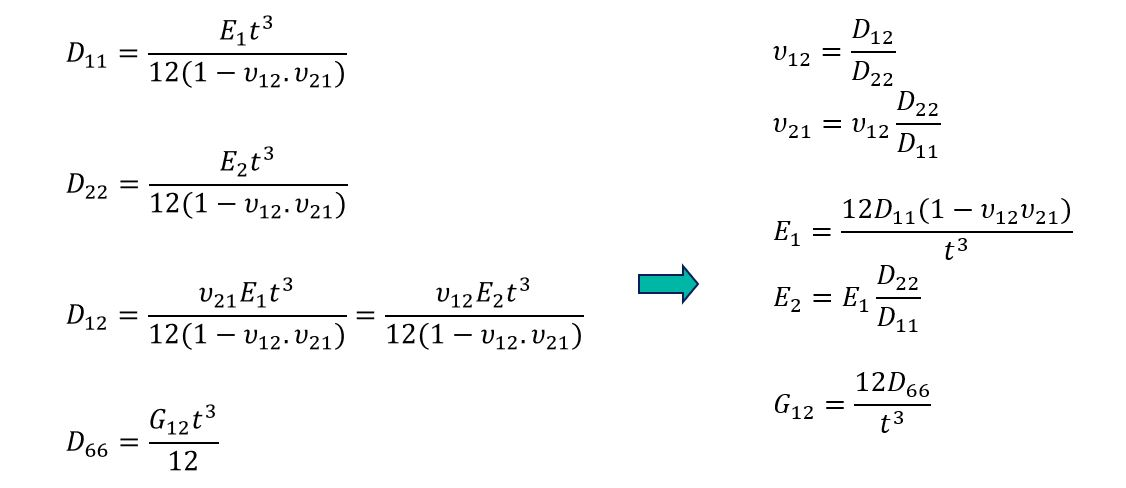#### Description: Multiplex wooden plate (7 layers 0°/90° of oak veneer)

The first step in the resonalyser procedure for the identification of orthotropic engineering constants is to define the correct aspect ratio for a “Poisson” plate. This information can be obtained by measuring the resonance frequencies of two beams cut along the plate edges. The beams cut along the 1-direction (Beam 1) and the 2-direction (Beam 2) have the following dimensions:

Beam 1:  Length 0.5995 m, Width 0.04711 m, Thickness 0.015 m, Mass 0.0.214 kg

Beam 2:  Length 0.549 m, Width 0.04709 m, Thickness 0.015 m, Mass 0.1958 kg

The measured resonance frequency for Beam 1 had a value of 141.7 Hz and for Beam 2 a value of 164.6 Hz.

With the above information, the aspect ratio for a Poisson plate could be computed as 1.013. The plate was cut according to this aspect ratio with the following dimensions:

Test plate (“Poisson” plate):  Length 0.55 m, Width 0.54 m, Thickness 0.015 m, Mass 2.255 kg

The 3 first measured resonance frequencies of this plate are:

1. Torsional Modal shape (measured in diagonal suspension – accelm. in node 73):   64.8 Hz
2. Saddle Modal shape (measured in vertical suspension- accelm. in node 77):           161.1 Hz
3. Breathing Modal shape (measured in diagonal suspension-accelm. in node 73):    173.3 Hz

The completed information of the general information screen is shown below.General data sheet of the Cross Oak plate

With this information, the resonalyser procedure can compute the orthotropic plate rigidities and thus the Engineering constants. The relationship between the plate rigidities and the engineering constants is shown below (t = plate thickness).The figure below shows a screenshot of the obtained results for the multiplex plate with the Engineering Constants E1 (Young’s Modulus in the 1-direction), v12 (Poisson’s ratio) and G12 (In-plane Shear Modulus), the plate rigidities D11, D22, D12 and D66 and uncertainty bounds on the identified plate rigidities.

The computed uncertainties on the plate rigidities (and hence on the Engineering constants) are small. This shows that the quality of the beams and plate is very good. The quality of the test beams and plate (and hence the uncertainty of the resonalyser results) is mainly determined by the homogeneity of the material and thickness.

A summary report of the data and the results is automatically stored in a .TXT File. The report file for this example is given below. It can be seen that the Experimental frequencies and the Numerical frequencies match well. This indicates that the quality of the test plate is good (homogeneous material properties all over the plate area)

Cross_Oak_Report